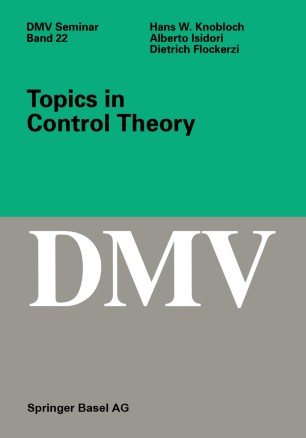# Topics in Control Theory

• Hans W. Knobloch
• Dietrich Flockerzi
• Alberto IsidoriBook

Part of the DMV Seminar book series (OWS, volume 22)

1. Front Matter
Pages i-vi
2. Hans W. Knobloch, Dietrich Flockerzi, Alberto Isidori
Pages 1-4
3. Hans W. Knobloch, Dietrich Flockerzi, Alberto Isidori
Pages 5-36
4. Hans W. Knobloch, Dietrich Flockerzi, Alberto Isidori
Pages 37-68
5. Hans W. Knobloch, Dietrich Flockerzi, Alberto Isidori
Pages 69-81
6. Hans W. Knobloch, Dietrich Flockerzi, Alberto Isidori
Pages 83-97
7. Hans W. Knobloch, Dietrich Flockerzi, Alberto Isidori
Pages 99-111
8. Back Matter
Pages 113-168

### Introduction

One of the key concerns in modern control theory is the design of steering strategies. The implementation of such strategies is done by a regulator. Presented here is a self-contained introduction to the mathematical background of this type of regulator design. The topics selected address the matter of greatest interest to the control community, at present, namely, when the design objective is the reduction of the influence of exogeneous disturbances upon the output of the system. In a first scenario the disturbance signal is regarded as a deterministic time series with known dynamics but unknown parameters. The design objective is then the asymptotic disturbance compensation. In a second scenario, no information about the disturbance signal is available apart from some bounds. Here, in an H-approach, control strategies are worked out which will prove efficient for all such disturbances. The intention of this book is to present ideas and methods on such a level that the beginning graduate student will be able to follow current research. New results are included, especially for nonlinear control systems, and as a service to the reader, an extensive appendix presents topics from linear algebra, invariant manifolds and calculus of variations, information which is hardly to be found in standard textbooks. Contents: Introduction • The problem of output regulation • Introduction • Problem statement • Output regulation via full information • Output regulation via full error feedback • A particular case • Well-posedness and robustness • The construction of a robust regulator • Disturbance attenuation via H-methods • Introduction • Problem statement • A characterization of the L2-gain of a linear system • Disturbance attenuation via full information • Disturbance attenuation via measured feedback • Full information regulators • Problem statement • Time-dependent control strategies • Examples • Time-independent control strategies • The local case • Nonlinear observers • Problem statement • Time-dependent observers • Error feedback regulators • Examples • Nonlinear H-techniques • Introduction • Construction of the saddle-point • The local scenario • Disturbance attenuation via linearization • Matrix equations • Linear matrix equations • Algebraic Riccati equations • Invariant manifolds • Existence theorem • Outflowing manifolds • Asymptotic phase • Convergence for T ¹ • A special case • Dichotomies and Lyapunov functions • Hamilton-Jacobi-Bellman-Isaacs equation • Introduction • Method of characteristics • The equation of Isaacs • The Hamiltonian version of Isaacs' equation • Bibliography

### Keywords

algebra calculus control control system control theory linear algebra nonlinear control

#### Authors and affiliations

• Hans W. Knobloch
• 1
• Dietrich Flockerzi
• 1
• Alberto Isidori
• 2
1. 1.Mathematisches InstitutUniversität WürzburgWürzburgGermany
2. 2.Dipartimento di InformaticaUniversità degli Studi di RomaRomaItaly

### Bibliographic information

• DOI https://doi.org/10.1007/978-3-0348-8566-9
• Copyright Information Birkhäuser Verlag 1993
• Publisher Name Birkhäuser, Basel
• eBook Packages
• Print ISBN 978-3-0348-9683-2
• Online ISBN 978-3-0348-8566-9
• Buy this book on publisher's site